# Data Mining FrequentItemset Mining Data Mining Some mining

• Slides: 13Data Mining, Frequent-Itemset Mining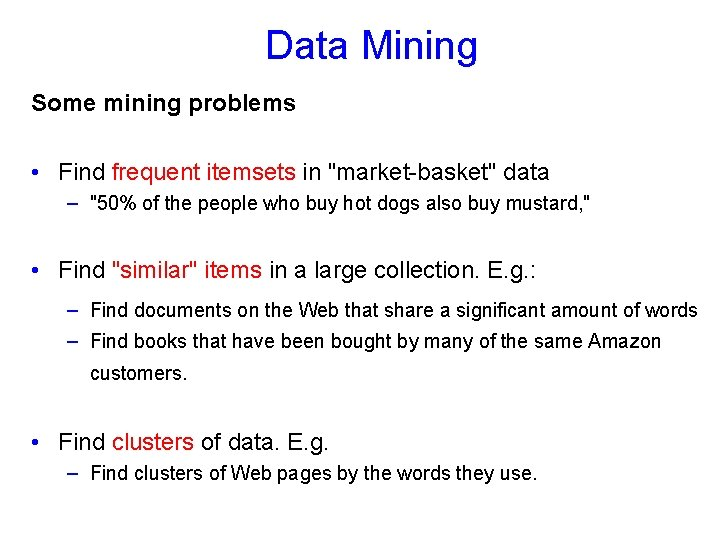Data Mining Some mining problems • Find frequent itemsets in "market-basket" data – "50% of the people who buy hot dogs also buy mustard, " • Find "similar" items in a large collection. E. g. : – Find documents on the Web that share a significant amount of words – Find books that have been bought by many of the same Amazon customers. • Find clusters of data. E. g. – Find clusters of Web pages by the words they use.Frequent-Itemset Mining Market-Basket Model • A large set of items, e. g. , things sold in a supermarket. • A large set of baskets, each of which is a small set of the items, e. g. , the things one customer buys on one day. Fundamental problem • What sets of items are often bought together? Application • If a large number of baskets contain both hot dogs and mustard, we can use this information in several ways. How?Beer and Diapers • What’s the explanation here?On-Line Purchases Amazon. com offers several million different items for sale, and has several tens of millions of customers. • Baskets = Customers, • Items = Books, DVDs, etc. • Motivation: Find out what items are bought together. • Baskets = Books, DVDs, etc. • Items = Customers • Motivation: Find out similar customers.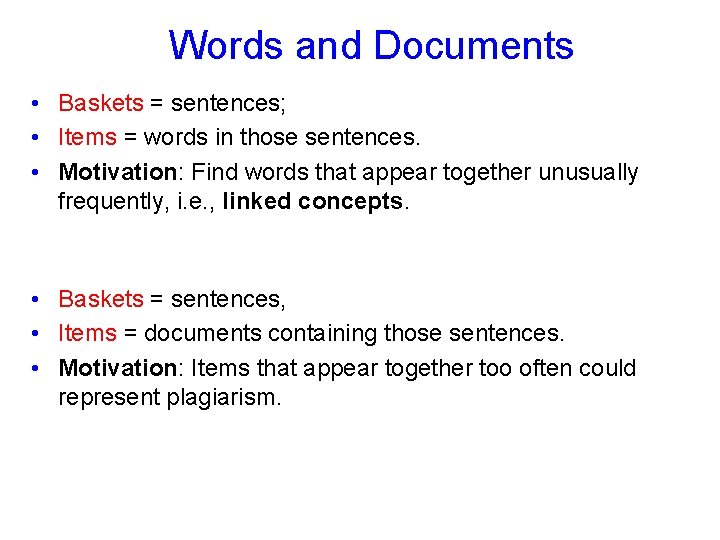Words and Documents • Baskets = sentences; • Items = words in those sentences. • Motivation: Find words that appear together unusually frequently, i. e. , linked concepts. • Baskets = sentences, • Items = documents containing those sentences. • Motivation: Items that appear together too often could represent plagiarism.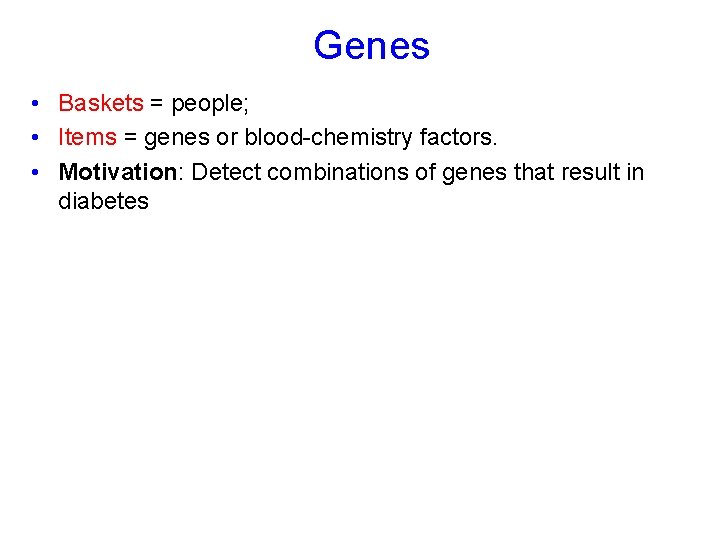Genes • Baskets = people; • Items = genes or blood-chemistry factors. • Motivation: Detect combinations of genes that result in diabetesSupport • Support for a set of items (itemset) I = the number of baskets containing all items in I. • Given a support threshold s, itemsets that appear in > s baskets are called frequent itemsets.Example: Frequent Itemsets • Items={milk, coke, pepsi, beer, juice}. • Support = 3 baskets. B 1 = {m, c, b} B 2 = {m, p, j} B 3 = {m, b} B 4 = {c, j} B 5 = {m, p, b} B 6 = {m, c, b, j} B 7 = {c, b, j} B 8 = {b, c} • Frequent itemsets: {m}, {c}, {b}, {j}, {m, b}, {b, c} , {c, j}.Scale of Problem • Wal. Mart sells 100, 000 items and can store billions of baskets. • The Web has over 100, 000 words and billions of pages.Association Rules • If-then rules about the contents of baskets. • {i 1, i 2, …, ik} → j means: “if a basket contains all of i 1, …, ik then it is likely to contain j. ” • Confidence of this association rule is the probability of j given i 1, …, ik. Example B 1 = {m, c, b} B 3 = {m, b} B 5 = {m, p, b} B 7 = {c, b, j} B 2 = {m, p, j} B 4 = {c, j} B 6 = {m, c, b, j} B 8 = {b, c} • An association rule: {m, b} → c. – Confidence = 2/4 = 50%.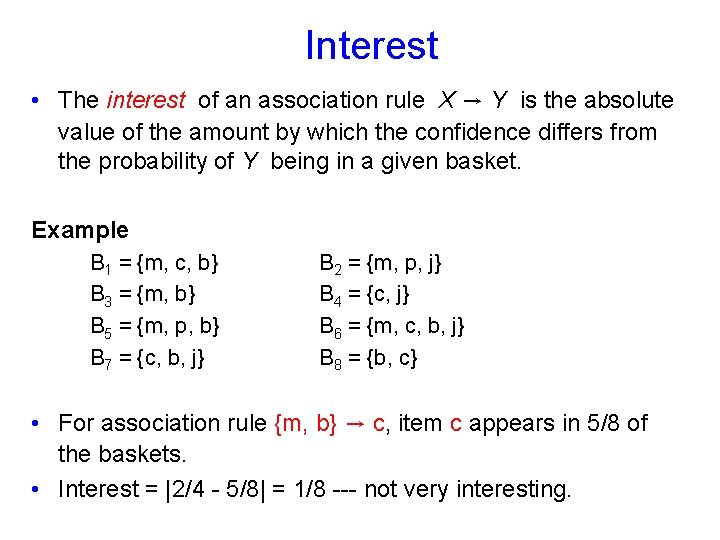Interest • The interest of an association rule X → Y is the absolute value of the amount by which the confidence differs from the probability of Y being in a given basket. Example B 1 = {m, c, b} B 3 = {m, b} B 5 = {m, p, b} B 7 = {c, b, j} B 2 = {m, p, j} B 4 = {c, j} B 6 = {m, c, b, j} B 8 = {b, c} • For association rule {m, b} → c, item c appears in 5/8 of the baskets. • Interest = |2/4 - 5/8| = 1/8 --- not very interesting.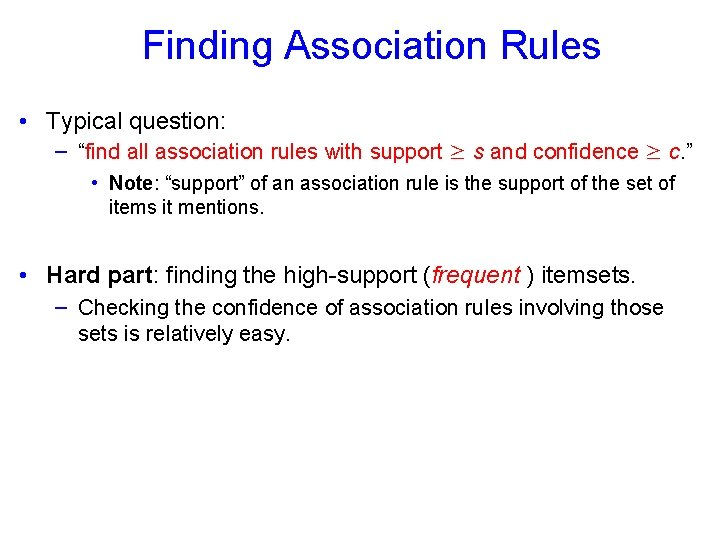Finding Association Rules • Typical question: – “find all association rules with support ≥ s and confidence ≥ c. ” • Note: “support” of an association rule is the support of the set of items it mentions. • Hard part: finding the high-support (frequent ) itemsets. – Checking the confidence of association rules involving those sets is relatively easy.